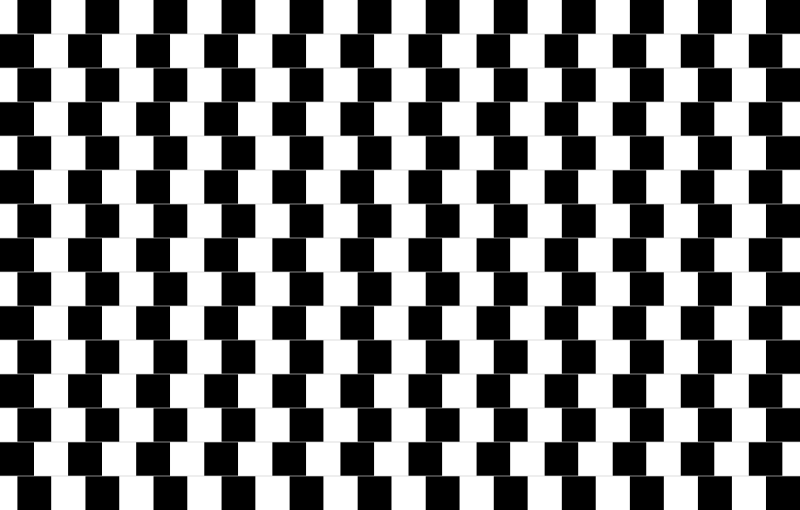#### Howdy, Stranger!

We are about to switch to a new forum software. Until then we have removed the registration on this forum.

# How would I make this checker pattern move left and right?

edited October 2017

I made a checker pattern with a while loop, and I want one set of loops to move 6px to the right and then back. I also have a second loop that I want to move 6px to the left and then back. I keep trying different things, but it keeps leaving a gap. The code I have so far is just the checker pattern, any help would be appreciated.

1. float g=0;
2. int g2=0;
3. int g3=0;
4. int g4=0;
5. float h=0;
6. int h2=0;
7. int h3=0;
8. int h4=0;
9. color c1=(#000000);
10. color c2=color(170,39,126);
11. color c3=color(27,136,132);
12. float n=0;
13. void setup()
14. {
15. size(800,800);
16. for(int i=0;i<height; i++)
17. {
18. color c=lerpColor(c1,c2,n);
19. stroke(c);
20. line(0,i,width,i);
21. n+=0.001;
22. }
23. }
24. void draw()
25. {
26. while(g<375){
27. checkers(g,h);
28. checkers(g-50,h+50);
29. checkers(g-100,h+100);
30. checkers(g-150,h+150);
31. checkers(g-200,h+200);
32. checkers(g-250,h+250);
33. checkers(g-300,h+300);
34. checkers(g-350,h+350);
35. g=g+50;
36. }
37. while(g2<350){
38. checkers(g2-25,h2+25);
39. checkers(g2-75,h2+75);
40. checkers(g2-125,h2+125);
41. checkers(g2-175,h2+175);
42. checkers(g2-225,h2+225);
43. checkers(g2-275,h2+275);
44. checkers(g2-325,h2+325);
45. g2=g2+50;
46. }
47. while(g3<850){
48. checkers(g3+400,h3+775);
49. checkers(g3+450,h3+725);
50. checkers(g3+500,h3+675);
51. checkers(g3+550,h3+625);
52. checkers(g3+600,h3+575);
53. checkers(g3+650,h3+525);
54. checkers(g3+700,h3+475);
55. checkers(g3+750,h3+425);
56. g3=g3+50;
57. }
58. while(g4<850){
59. checkers(g4+475,h4+750);
60. checkers(g4+525,h4+700);
61. checkers(g4+575,h4+650);
62. checkers(g4+625,h4+600);
63. checkers(g4+675,h4+550);
64. checkers(g4+725,h4+500);
65. checkers(g4+775,h4+450);
66. g4=g4+50;
67. }
68. }
69. void checkers(float g, float h)
70. {
71. noStroke();
72. fill(0);
73. rect(0+g,0+h,25,25);
74. fill(255);
75. rect(25+g,0+h,25,25);
76. }
Tagged:

• I see two chess boards cut in half

You wrote:

one set of loops to move 6px to the right

Do you want to move the entire chess board? Or just one line / one pair?

And when do you want this to happen, when you press the mouse?

• a general chess board is done like this. Your way is much to complicate

``````int cellSizeX = 25;
int cellSizeY = 25;

void setup() {
size(600, 600);
background(255);
fill(0);
} // func

void draw() {
background(255);

for (int x = 0; x < 15; x++) {
for (int y = 0; y < 15; y++) {

// if cell is true
if ( isEven(x) && !isEven(y) ||
!isEven(x) && isEven(y) )
fill(255, 2, 2); // red
else
fill(1, 2, 255);  // blue

rect(x*cellSizeX, y*cellSizeY,
cellSizeX-1, cellSizeY-1);
//
} // for
} // for
//
} // func

boolean isEven(int n)
{
return n%2 == 0;
}
//
``````
• Your way is still too complicated 8)

Square colour can be calculated on the sum of x and y...

``````if ( isEven(x + y) ) {
...
}
``````
• So essentially I want to set an idle animation that will move g and g3 to the right 6px and then move it back. Also idle, moving g2 and g4 to the left and move back. I pretty much want to do this.• so do you want the change instantaneously or animated from 0 to 6 ?

set the offsetX to 6. You can use function `void mousePressed()` and say

``````if(offsetX == 6)
offsetX  =0;
else
offsetX  =6;
``````

to toggle between two situations

• I guess animated, and I would like to have it do this without me having to interact (clicking, keys, ect.).

• ``````offsetX+=offsetXAdd;

if(offsetX>=6)

if(offsetX<=-6)
``````
• Would offsetX be a float?

• yes

• I am also a complete novice at code, and I have no clue where to place this to make it work. I also appreciate you working with me on this.

• you need to add offsetX to the lines in the grid you want to move

• So if had this from the previous code

1. while(g<375){
2. checkers(g,h);
3. checkers(g-50,h+50);
4. checkers(g-100,h+100);
5. checkers(g-150,h+150);
6. checkers(g-200,h+200);
7. checkers(g-250,h+250);
8. checkers(g-300,h+300);
9. checkers(g-350,h+350);
10. g=g+50;
11. }

Would I add the whole thing, part of it before the while, after the while, or after the whole thing. Sorry, I tried several ways, and it doesn't move.

2. if(offsetX>=6)
4. if(offsetX<=-6)
• edited October 2017 Answer ✓

It can really help conceptually do understand that you are trying to do a single bouncing ball, learn how to do that, then apply it to a bunch of objects so that they all bounce in the same way at the same time.

``````/**
* SimpleBounce
* Bounce a shape back and forth along a flat line
* 2017-10-15 Jeremy Douglass - Processing 3.3.6
* based on processing.org/examples/bounce.html
* see forum.processing.org/two/discussion/24554/how-would-i-make-this-checker-pattern-move-left-and-right
*/
float xstart;         // starting position of shape
int   xmax = 12;      // maximum distance from starting position
float xpos;           // current position of shape
float xspeed = 1;     // speed of shape
int   xdirection = 1; // currently moving left or right

void setup() {
size(200, 200);
frameRate(30);
rectMode(CENTER);
// Set the starting position and current position of the shape
xstart = width/2;
xpos = xstart;
}

void draw() {
background(192);
// Update the position of the shape
xpos = xpos + ( xspeed * xdirection );
// Test to see if the shape exceeds the boundaries
if (xpos > xstart + xmax || xpos < xstart - xmax) {
// If it does, reverse its direction by multiplying by -1
xdirection *= -1;
}

// Draw the shape at its current position
rect(xpos, height/2, 50, 50);
}
``````
• I agree with jeremy.

also, in this section you want `checkers(g+offsetX,h);`